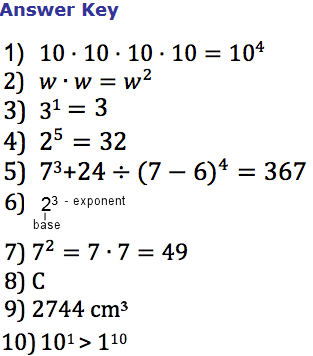Lesson - Powers and Exponents

Powers and Exponents

Key Terms

A power includes a base number and an exponent.

An exponent shows us how many times the base needs to be used as a factor.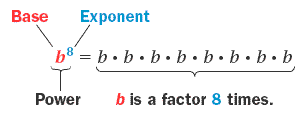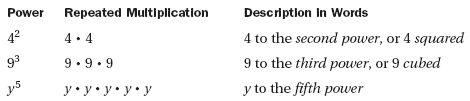How to Evaluate a Power

Example

Evaluate five cubed.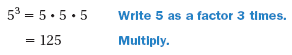Write the product as a power.

1)    10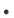101010

Write the product as a power.

2)    ww

Describe the power in words and then evaluate.

3)     31

Describe the power in words and then evaluate.

4)     25

Order of Operations

When you evaluate expressions with powers, evaluate any powers before multiplying or dividing.

1.  Evaluate expressions inside grouping symbols.

2.  Evaluate powers.

3.  Multiply and divide from left to right.

4.  Add and subtract from left to right.

Using a Power

Example

To find the height of a cliff, use the expression 16t2.  This expression gives the distance in feet that an object has fallen t  seconds after it begins to fall. If an object takes 5 seconds to fall from the top of the cliff, what is the height of the cliff?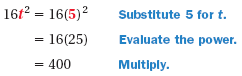Answer:  The height of the cliff is 400 feet.

Using the Order of Operations

Example 1

Evaluate the expression.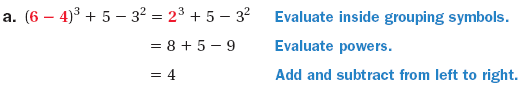Example 2

Evaluate the expression.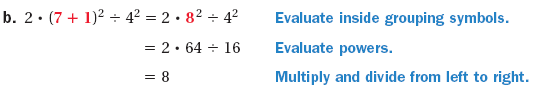5)     Evaluate the expression.

3 + 24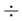(7 - 6) 4

6)     Write a power in the space below and label the base and the exponent.

7)    Describe and correct the error in the solution given below.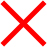2 = 7 x 2

= 14

8)    What is the value of the expression 53 - 3

A.
B.
19
C.
44
D.   128

9)     The volume of a cube is s3, where s is the length of one side of the cube.  A cube has a side length of 14 centimeters.  What is the volume of the cube in cubic centimeters?

A.  42
B.  196

C.  1400

D.  2744

10)  Complete the statement using  <,  >,  or  =.

101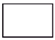110